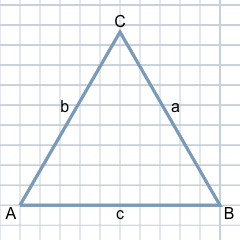# Calculate Equilateral Triangle

Compared to general triangles, an equilateral triangle is a special type of triangle because it has three sides of exactly the same length. In the following section, we will use an example with a given side length to calculate the area, the perimeter, the angles and the heights for the equilateral triangle. To do this, we will use the formulas and calculation rules that have been simplified specifically for equilateral triangles.

On the page of our Triangle Calculator, you will find a lot of information on calculating not only equilateral but also general triangles. Alternatively, visit our guides on the topics 'Area of a Triangle' and 'Right-angled Triangles'.

## Definition of an Equilateral TriangleBefore we go into the calculation of equilateral triangles in more detail, here is a brief definition of the equilateral triangle.

Basically, a triangle is defined by three points in the plane that do not lie on a straight line. The three points form the corners of the triangle. Each connecting line between two such corners is a side of the triangle. In the plane, the triangle therefore delimits a surface.

The special feature of the equilateral triangle compared to the general triangle is that all three sides of the triangle have the same length, as the figure shown here illustrates.

By default, the clockwise corners are labelled with the uppercase letters A, B, C. The sides opposite these corners are given the corresponding lowercase letters a, b and c. In the case of an equilateral triangle, all three sides are usually labelled a.

## Introduction to the Calculation of Equilateral Triangles

Equilateral triangles differ from general triangles only in that all three sides have the same length. Basically, a triangle can be unambiguously determined if all three sides a, b and c are known. Therefore, it is sufficient to calculate an equilateral triangle if only one side is known.

However, in the case of the equilateral triangle with a given side a, simpler calculations are also possible. For example, all three angles in the equilateral triangle are also always equal and always amount to 60 degrees. Likewise, the calculation of the area of an equilateral triangle follows a simpler formula than, for example, in the case of the general triangle with three given sides a, b and c, as we will see below.

## Example for the calculation of an equilateral triangle

Below we show an example of the calculation of an equilateral triangle where the side length a is known. Using this one given value, the other properties of the equilateral triangle can now be clearly determined step by step.

#### Example

Let the side length a = 5 in be given. Since it is an equilateral triangle, the value of all three sides is already known.

#### Explanation

We are looking for the area, the perimeter, the angles and the heights of all three sides of the equilateral triangle.

You can enter the given value for side a into the equilateral triangle calculator after selecting "One side for an equilateral triangle" under "What values are given?". The calculator will then calculate, as in the following calculations, all the values sought for the triangle and will also output a graphical result of the calculated triangle.

### How is the area in an equilateral triangle calculated?

In an equilateral triangle, as the name implies, all three sides are of equal length. Therefore, the following simplified formula applies to the area of the equilateral triangle, where a is the length of one of the sides

A = 3 / 4 × a²

### Inserting the existing value for the page length

If you insert the value for a, you get

A = 3 / 4 × 5² ≈ 10.83 in²

### Solution

The area A of the equilateral triangle is 10.83 in².

### How is the perimeter of an equilateral triangle calculated?

Using the given length for one side and thus all three sides, the perimeter of the triangle can be determined as follows:

### Formula: Perimeter P of an equilateral triangle

The perimeter of each triangle is the sum of the lengths of all three sides a, b and c.

P = a + b + c

### Inserting the existing value

If we insert the given value a = 5, which is valid for each of the three sides, we get

P = 5 + 5 + 5 = 15

### Solution

The perimeter of the equilateral triangle is 15 in.

### Solution

In each equilateral triangle, all angles are equal and each is 60°.

### How are the heights in the equilateral triangle calculated?

To calculate the height of a in the equilateral triangle, the following formula can be used instead of the more complicated formula for general triangles:

ha = 3 / 2 × a

### Inserting the existing values

Substituting the known value for a = 5 in, we obtain

ha = 3 / 2 × 5 ≈ 4.33

### Solution

The height of a, i.e., ha is 4.33 in.

Since sides b and c are exactly as long as a, their heights are also identical to a.

### What does the calculated equilateral triangle look like?

Thus the calculated equilateral triangle with given side a = 5 in can be drawn using all calculated values as follows:

### Graphical result1 box equals 0.5 units (as in the arithmetic book)

## Source information

As source for the information in the 'Triangle' category, we have used in particular:

## Last update on February 20, 2023

The pages of the 'Triangle' category were last editorially reviewed by Michael Mühl on February 20, 2023. They all correspond to the current status.

### Previous changes on November 12, 2022

• 12.11.2022: Publication of an article Calculation of equilateral triangles.
• 12.11.2022: Publication of an article about Area of a triangle and about Right-angled triangles.
• 12.11.2022: Publication of the topic Calculate Triangle together with the corresponding texts.
• Editorial revision of all texts in this category